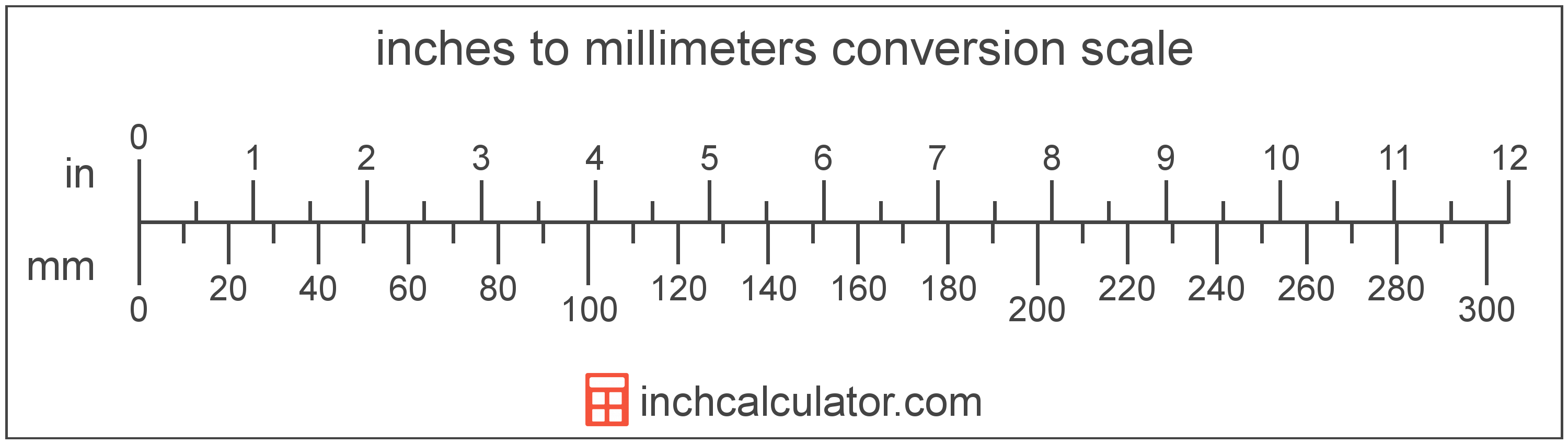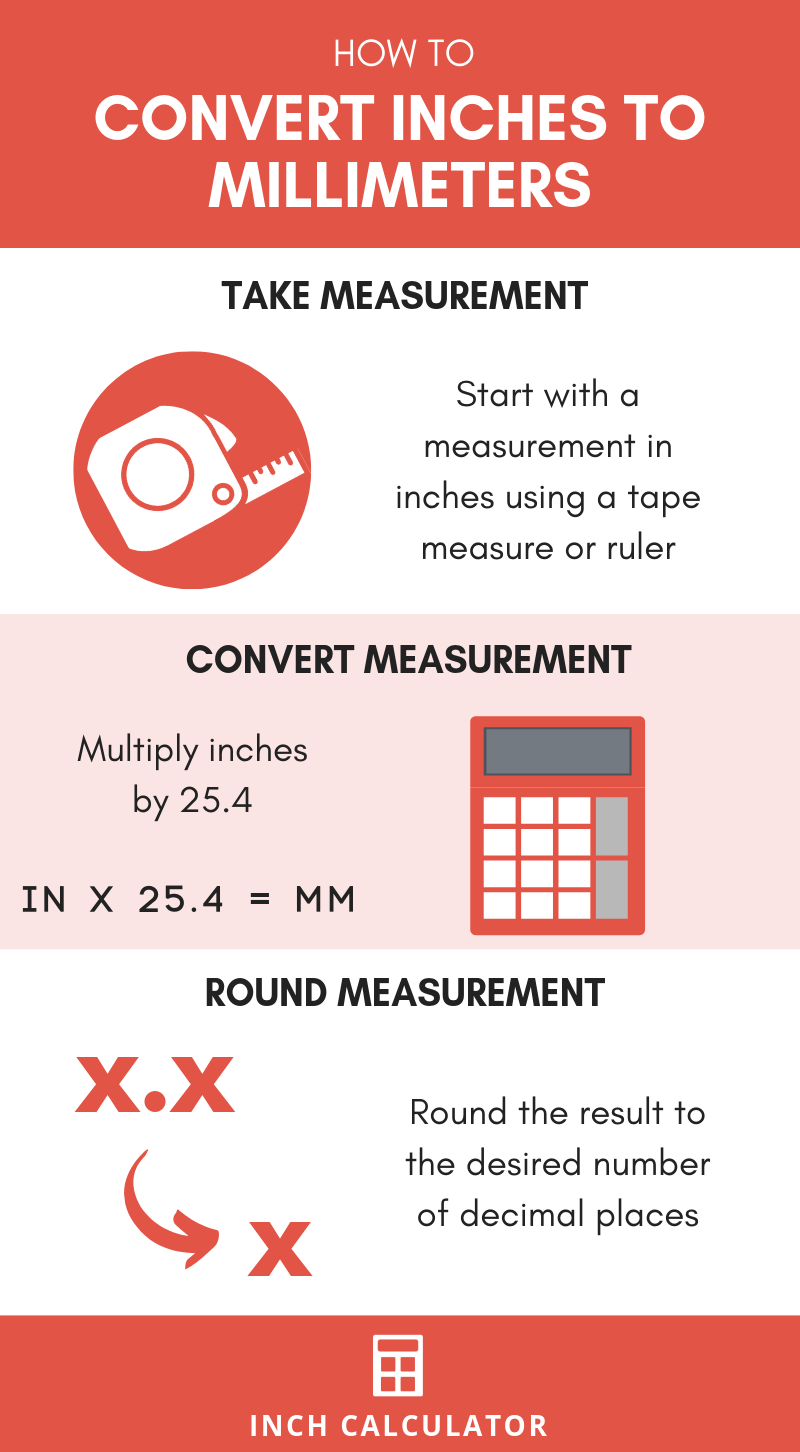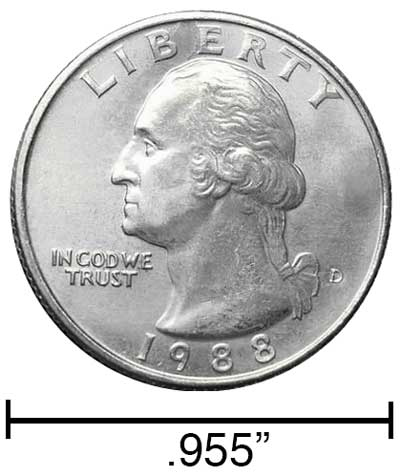# Inches to Millimeters Conversion Calculator

Enter the length in inches below to get the value converted to millimeters.

/
Results in Millimeters:1 in = 25.4 mm
1 in = 2.54 cm
Do you want to convert mm to inches?

## How to Convert Inches to MillimetersThe fastest and easiest way to convert inches to mm (millimeters) is use this simple formula:

millimeters = inches × 25.4

Since there are 25.4 millimeters in one inch, the length in millimeters is equal to inches times by 25.4. Thus, the formula to convert inches to millimeters is the length multiplied by 25.4.

For example, here's how to convert 5 inches to millimeters using the formula above.
5" = (5 × 25.4) = 127 mm

### How Many Millimeters are in an Inch?

There are 25.4 millimeters in an inch, which is why we use this value in the formula above.

1" = 25.4 mm

Our inch fraction calculator can add inches and millimeters together and it also automatically converts the results to US customary, imperial and SI metric values.## What is an Inch?

An inch is a unit of linear length measure equal to 1/12 of a foot or 1/36 of a yard. Because the international yard is legally defined to be equal to exactly 0.9144 meters, one inch is equal to 2.54 centimeters.

The inch is a US customary and imperial unit of length. Inches can be abbreviated as in; for example, 1 inch can be written as 1 in.

Inches can also be denoted using the symbol, otherwise known as a double-prime. Often a double-quote (") is used instead of a double-prime for convenience. A double-prime is commonly used to express 1 in as 1″.The standard ruler has 12", and is a common measuring tool for measuring inches. They are also often measured using tape measures, which commonly come in lengths from 6' - 35'. Other types of measuring devices include scales, calipers, measuring wheels, micrometers, yardsticks, and even lasers.

## What is a Millimeter?

One millimeter is equal to one-thousandth (1/1,000) of a meter, which is defined as the distance light travels in a vacuum in a 1/299,792,458 second time interval.

The millimeter, or millimetre, is a multiple of the meter, which is the SI base unit for length. In the metric system, "milli" is the prefix for 10-3. Millimeters can be abbreviated as mm; for example, 1 millimeter can be written as 1 mm.

Millimeters are often represented by the smallest ticks on most metric rulers. To get a reference point of the size, the thickness of a US dime is 1.35mm.

We recommend using a ruler or tape measure for measuring length, which can be found at a local retailer or home center. Rulers are available in imperial, metric, or combination with both values, so make sure you get the correct type for your needs.

Need a ruler? Try our free downloadable and printable rulers, which include both imperial and metric measurements.

## Inch to Millimeter Conversion Table

Inch measurements converted to millimeters
Inches Millimeters
1" 25.4 mm
2" 50.8 mm
3" 76.2 mm
4" 101.6 mm
5" 127 mm
6" 152.4 mm
7" 177.8 mm
8" 203.2 mm
9" 228.6 mm
10" 254 mm
11" 279.4 mm
12" 304.8 mm
13" 330.2 mm
14" 355.6 mm
15" 381 mm
16" 406.4 mm
17" 431.8 mm
18" 457.2 mm
19" 482.6 mm
20" 508 mm
21" 533.4 mm
22" 558.8 mm
23" 584.2 mm
24" 609.6 mm
25" 635 mm
26" 660.4 mm
27" 685.8 mm
28" 711.2 mm
29" 736.6 mm
30" 762 mm
31" 787.4 mm
32" 812.8 mm
33" 838.2 mm
34" 863.6 mm
35" 889 mm
36" 914.4 mm
37" 939.8 mm
38" 965.2 mm
39" 990.6 mm
40" 1,016 mm

## References

1. National Institute of Standards and Technology, SI Units - Length, https://www.nist.gov/pml/weights-and-measures/si-units-length
2. National Institute of Standards and Technology, Checking the Net Contents of Packaged Goods, Handbook 133 - 2019 Edition, https://nvlpubs.nist.gov/nistpubs/hb/2019/NIST.HB.133-2019.pdf
3. United States Mint, Coin Specifications, https://www.usmint.gov/learn/coin-and-medal-programs/coin-specifications